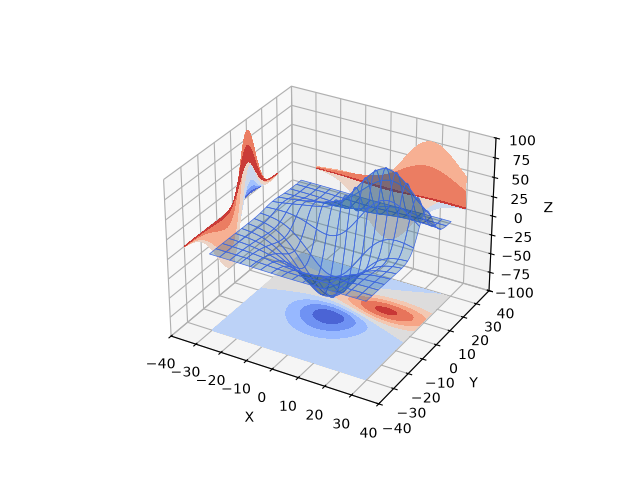# Project filled contour onto a graph#

Demonstrates displaying a 3D surface while also projecting filled contour 'profiles' onto the 'walls' of the graph. See Project contour profiles onto a graph for the unfilled version.import matplotlib.pyplot as plt

from mpl_toolkits.mplot3d import axes3d

X, Y, Z = axes3d.get_test_data(0.05)

# Plot the 3D surface
ax.plot_surface(X, Y, Z, edgecolor='royalblue', lw=0.5, rstride=8, cstride=8,
alpha=0.3)

# Plot projections of the contours for each dimension.  By choosing offsets
# that match the appropriate axes limits, the projected contours will sit on
# the 'walls' of the graph
ax.contourf(X, Y, Z, zdir='z', offset=-100, cmap='coolwarm')
ax.contourf(X, Y, Z, zdir='x', offset=-40, cmap='coolwarm')
ax.contourf(X, Y, Z, zdir='y', offset=40, cmap='coolwarm')

ax.set(xlim=(-40, 40), ylim=(-40, 40), zlim=(-100, 100),
xlabel='X', ylabel='Y', zlabel='Z')

plt.show()


Gallery generated by Sphinx-Gallery